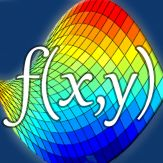Português

Todos os dias oferecemos software com licenças GRATUITAS que do contrário você teria que comprar!\$1.99

# iPhone Giveaway of the Day - VisualMATH 4D

VisualMATH 4D is a graphical calculator and lets you draw graphs of your mathematical functions in 2D, 3D and 4D.

This giveaway offer has been expired. VisualMATH 4D is now available on the regular basis.

VisualMATH 4D is a graphical calculator and lets you draw graphs of your mathematical functions in 2D, 3D and 4D with a time-variable (t).

Features:

- draw graphs with one or two variables like f(x), f(x,y)
- visualize your function in 4 dimensions with a time-variable
- compute value tables
- definite integral and derivative - functions
- matrices, vectors, determinante, inverse, transpose,
- trigonometrical functions like sin, cos, tan, sec
- rounding-functions, floor, ceil, round,
- sum and product-function
- save your functions and make screenshots
- modify your graph-range and set customized color options
- rotate and zoom your graph in three dimensions
- display multiple graphs

The app is easy to use and helps students and engineers to visualize their mathematical functions and it can be used as a professional graphical calculator.

Ronny Weidemann

Education

4.1

16.6 MB

4+

### Idiomas:

English